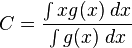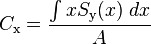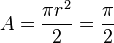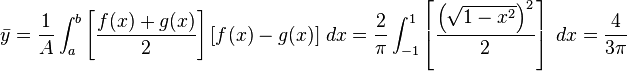# The Magic of Balance

CalTech sent me a postcard early in the fall asking a question: how do you balance a glass of water that’s tilted at an angle. I thought about that problem back then but couldn’t come up with a solution quickly, so I just gave up. Today, as I was searching for independent projects on Google, I stumbled upon the Wiki How website for “How to balance a soda can at a 45 degree angle” (http://www.wikihow.com/Balance-a-Soda-Can-at-a-45-Degree-Angle)(image from the wikihow website)

According to the wiki how website, if you pour out around half of the soda in the can, and then tilt it to about 45 degrees, it is possible to balance the can on the table. It seems really cool and magical, so I decided to pick up this old problem from the postcard and look into how we can balance a tilted glass or soda can.

We know that the key of balancing things is to find the center of mass (or center of gravity) of the object. For a single rigid object with uniform density, the center of mass is located at the centroid. Therefore, in order to find the center of mass, we first need to learn to find the centroid.

Centroid

Definition from Wikipedia: “The centroid of a two-dimensional shape X is the intersection of all straight lines that divide X into two parts of equal moment about the line. It can be calculated as the arithmetic mean (informally, the “average”) of all points of X. The definition extends to any object X in n-dimensional space: its centroid is the intersection of all hyperplanes that divide X into two parts of equal moment.”

The calculus way of finding the centroid of a shape uses the integral:This equation reminds us of the joint probability density function that we learned just before spring break. In fact, since the centroid is defined as the “arithmetic mean of all points of shape X”, we are using a joint density function to define the position of the centroid.

With multiple coordinates, we use a similar equation:(where Ck is the kth coordinate of C, and Sk(z) is the measure of the intersection of X with the hyperplane defined by the equation xk = z.)

So for a plane, we find the coordinates from these equations:Wikipedia gives an example of finding the centroid of a semicircle bounded byand. Its area isTherefore the coordinates of the centroid are.

For a 3-D object, we can find the x, y and z coordinates of the centroid by using this method.

So that concludes step one — finding the center of mass for an object with a uniform density. The next question would be: “How do you take density into account?” The glass of water obviously has two different substances with different density, and finding its centroid is going to be much harder. I’d like to look further into this problem in the future!

Just a couple more interesting pictures of magically balanced objects!(From reference #3 website)
References:
http://en.wikipedia.org/wiki/Center_of_mass
http://en.wikipedia.org/wiki/Centroid#By_integral_formula
https://webspace.utexas.edu/cokerwr/www/index.html/science25.html
http://www.wikihow.com/Balance-a-Soda-Can-at-a-45-Degree-Angle
Tagged with: , , , , , , , ,
Posted in Multivariable Calculus
###### 5 comments on “The Magic of Balance”
1.harveyjohnson says:

Excellent Zfu71! Good synopsis and presentation of your solution! I can’t wait to see how you deal with objects of different densities!

•zfu71 says:

tried it out on a soda can and miserably failed… hmm maybe this is more theoretical than practical…

•harveyjohnson says:

How cool… I hope you didn’t lose a lot of soda.. Maybe we need to figure out how you can distinguish between stable equilibrium points and unstable equilibrium points.

2.zfu71 says:

Reblogged this on Fu.

3. […] from my last post, I further researched into the calculation for center of mass. Today’s post is going to be […]

Popular